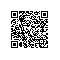# C控制台实现模拟平抛运动算法

#include <stdio.h>
#include <conio.h>
#include <stdlib.h>
#include <string.h>
#include <windows.h>
#include <fcntl.h>

int main(void)
{
float v0 = 0.01 ;
float g = 9.8 , t , x , s = 0.0, y;
int j ,k , temp;
for(t = 0 ; t < 10 ; t++)
{
x = v0 * t ;
y = 0.5*g*t*t;
s = 0.5*g*t*t;
if(s != 0){
printf("*->x:%.2f y:%.2f",x,y);
temp = t ;
while(temp--)
printf("\n");
printf("|");
Sleep(1000);
}
for(j = 0 ; j < t ; j++){
printf("       ");
}
}
return 0 ;
}使用钉钉扫一扫加入圈子
+ 订阅